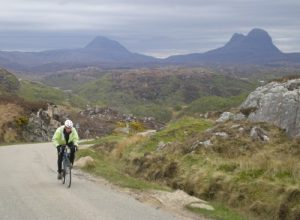The kinetic energy of a drifting tectonic plate…

…is broadly similar to the kinetic energy of me and my bike as I pedal along.

According the the theory of plate tectonics, the outer layer of the Earth is divided into a number of separate plates, which very slowly drift around, opening and closing oceans, causing earthquakes, and thrusting up mountain ranges.

A moving body has energy by virtue of its motion: kinetic energy. Kinetic energy is proportional to a body’s mass and to the square of its speed.

Now tectonic plates move extremely slowly: the usual comparison is with a growing fingernail. But they are also extremely heavy: tens of millions of square kilometres in area, over 100 km thick, and made of rock. I wondered how the minute speed and colossal mass play out against each other: what’s the kinetic energy of a drifting tectonic plate?

There are so many variables, that vary such a lot, that this calculation is going to be extremely approximate. But the answer is delightfully small: the kinetic energy of the tectonic plate on which I live, as observed from one of the plates next door, is about the same as the kinetic energy of me and my bike when I’m going at a reasonable pace: about 1500 joules.Me struggling up one of the many steep roads in north-west Scotland. Here, the kinetic energy of me and my bike is much less than the kinetic energy of a drifting tectonic plate. In fact the speed of me and my bike is probably much less than that of a drifting tectonic plate ;-).

This is a fun calculation to do, but we shouldn’t get carried away thinking about the kinetic energy of tectonic plates. Plates are driven by huge forces, and their motion is resisted by equally large forces. The mechanical work done by and against these forces will dominate a plate’s energy budget in comparison to its kinetic energy.

But the calculation does provoke an interesting thought about forces and motion. I can get my bike up to full speed in, say, 10 seconds. If the Eurasian plate were as free to move as my bike, and I were to put my shoulder against it and shove as hard as I could, it would take me about 500 years to get it up to its (very tiny) full speed.

In both cases, I’m giving the moving object roughly 1500 joules of kinetic energy. How come I can give that energy to my bike in a few seconds, but to give it to the plate would take me centuries?

The calculation

Depending on how you count them, there are 6-7 major tectonic plates, 10 minor plates, and many more microplates. The plates vary hugely in size, from the giant Pacific Plate with an area of 100 million km2, to the dinky New Hebridean plate, which is a hundred times smaller. The microplates are smaller still. Plates also vary a lot in speed: 10-40 mm is typical.

I’m going to be parochial, and choose the Eurasian plate for this calculation.

Let’s call the area of the plate a and its mean thickness t. Its volume is then given by at, and if its mean density is ρ, then its mass m is ρat.

A body of mass m moving at a speed v has kinetic energy ½mv2. So our plate will have kinetic energy ½ρatv2.

The area of the Eurasian plate is 67,800,000 km2 or 6.78 × 1013 m2, and its speed relative to the African plate is (the only speed I have) is given as 7-14 mm per year. We’ll use 10 mm per year, which is 3.2 × 10-10 ms-1. The thickness of tectonic plates in general varies roughly in the range 100-200 km depending upon whether we are talking about oceanic or continental lithosphere; let’s call it 150 km or 1.5× 105 m. The density of lithospheric material varies in the range 2700-2900 kg m-3; we’ll use 2800 kg m-3.

Putting all of these numbers into our formula for kinetic energy, we get a value of 1500 joules (to 2 significant figures, which the precision of the input data certainly doesn’t warrant).

Now for me and my bike. I weigh about 57 kg, my bike is probably about 10 kg. Suppose I’m riding at 15 mph, which is 6.7 ms-1. My kinetic energy is almost exactly…

…1500 joules!

The closeness of these two values is unmitigated luck*, and we shouldn’t be  seduced by the coincidence. Just varying the speed of the plate in the range 7-14 mm would cause a 4-fold change in kinetic energy, and there’s the variability in plate thickness and rock density to take into account as well. The choice of bike speed was arbitrary, I guessed the mass of the bike, and I’ve since realised that I didn’t account for the fact that the wheels of my bike rotate as well as translate.

However, what we can say is that the kinetic energy of a drifting continent is definitely on a human scale, which leads to a new question:

Suppose the Eurasian plate were as free to move as my bicycle, and that I put my shoulder against it and shoved, how long would it take me to get it up to speed?

From the figures above, the mass of the plate is 2.85 × 1022 kg. If I can push with a force equal to my own weight (about 560 newtons) then by Newton’s 2nd Law I can give it an acceleration of about 1.96 × 10-20 ms-2. Rearranging the equation of motion v = at, where v is the final speed, a is the acceleration, and t is the time, then t = v/a. Inserting the values for v and a, we get t = 1.6 × 1010 seconds, or about 500 years.

* I didn’t tweak my assumptions: what you see above really is the very first version of the calculation!